• SCI论文写作中折线图和柱状图的区别在SCI论文写作中，描述实验数据的时候我们常常会遇到选择使用柱状图还是折线图的问题。那么我们应该如何选择呢？首先我们必须清楚的是，折线图的横坐标必须是有递减关系的，比如...编者按
科研人SCI由洪堡学者，香港大学，中科院等多单位的博士团队联合打造，专注于科研论文写作和科研咨询，欢迎大家点击上方蓝字科研人SCI关注我们。
SCI论文写作中折线图和柱状图的区别在SCI论文写作中，描述实验数据的时候我们常常会遇到选择使用柱状图还是折线图的问题。那么我们应该如何选择呢？首先我们必须清楚的是，折线图的横坐标必须是有递减关系的，比如时间的变化，浓度的变化，温度的变化等等。也就是说明纵坐标Y值是随着横坐标X的一种变化趋势，是不同时间点(温度等)的结果的连线。折线图的目的是为了让读者非常清楚的看到实验结果随着某个参数变化的趋势和走向。为的是突出和直观显示出某个重要参数对结果的影响。而柱状图的横坐标是相互独立的，比如几组独立的对照实验，不同样品之间的比较，与其他文献结果的比较等。
也就是说明
纵坐标Y
值是随着相对独立的横坐标X的一个结果，不同Y值没有相互的必然联系和递进关系
，是不同的实验结果
。
柱状图的目的是为了让读者非常清楚的对比不同实验的结果。为的是突出和直观显示出某个实验结果的优越性。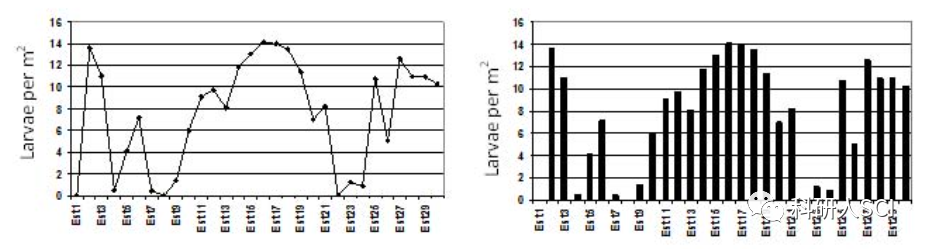图片来源：https://www.elsevier.com/connect/11-steps-to-structuring-a-science-paper-editors-will-take-seriously
科研人SCI
免费
为大家提供大量SCI写作干货和写作课程，有需要的小伙伴可以咨询编辑(微信号：keyanren2020)。同时欢迎大家加入科研人SCI学术交流群，助力科研，共同进步！(扫码添加小编微信拉您入群)
分享点赞加在看，来个【一键三连】吧 将科研人SCI分享给更多有需要的人
↓↓↓↓


展开全文• Python matplotlib：使用matplotlib绘制--柱状图和折线图的组合。废话不多说，直接上效果图和代码。代码如下:#柱状图import numpy as npimport matplotlib.pyplot as pltC1 = '#99CCFF'#C2 = '#CCFFFF'C2 = '#CCCCFF...
Python matplotlib：使用matplotlib绘制--柱状图和折线图的组合。
废话不多说，直接上效果图和代码。代码如下:
#柱状图
import numpy as np
import matplotlib.pyplot as plt
C1 = '#99CCFF'
#C2 = '#CCFFFF'
C2 = '#CCCCFF'
C3 = "#6699CC"
AAA = [227.74,165.24,146.42]
BBB = [234.78,188.48,158.94]
CCC = [284.83,200.33,162.23]
#CNN_RLSTM = [0.9016,0.8636,0.9435]
#x = ['REST','LAPT','AUTO']
x = np.arange(3) #总共有几组，就设置成几，我们这里有三组，所以设置为3
total_width, n = 0.8, 3 # 有多少个类型，只需更改n即可，比如这里我们对比了四个，那么
#就把n设成4
width = total_width / n
x = x - (total_width - width) / 2
'''
#plt.bar用于绘制柱状图
plt.bar(x, AAA, color = C2,width=width,label='AAA ') ###或者color C2可以换成默认
plt.bar(x + width, BBB, color = C1,width=width,label='BBB')
plt.bar(x + 2 * width, CCC , color = C3,width=width,label='CCC')
'''
###或者color C2可以换成默认
plt.bar(x, AAA, color = 'r',width=width,label='AAA ') ###或者color C2可以换成默认
plt.bar(x + width, BBB, color = 'y',width=width,label='BBB')
plt.bar(x + 2 * width, CCC , color = 'k',width=width,label='CCC')
#plt.bar(x + 3 * width, CNN_RLSTM , color = "g",width=width,label='CNN-RLSTM')
plt.xlabel("LUT")
plt.ylabel("Energy(KWh)")
plt.legend(loc = "best")
plt.xticks([0,1,2],['0.1','0.2','0.3'])
my_y_ticks = np.arange(120, 300, 50)
plt.ylim((150, 300))
plt.yticks(my_y_ticks)
AAA_plot = [2*0.16325,2* 0.13622, 2*0.11936]
BBB_plot = [2*0.1752, 2*0.14554,2*0.13491]
CCC_plot = [2*0.15599, 2*0.15473, 2*0.1956]
ax2 = plt.twinx() # 用于绘制双Y轴，重点。
'''
ax2.plot(x + width, AAA_plot, color=C2)
ax2.plot(x + width, BBB_plot, color=C1)
ax2.plot(x + width, CCC_plot, color=C3)
'''
# 折线也可换颜色
ax2.plot(x + width, AAA_plot, color='r')
ax2.plot(x + width, BBB_plot, color='y')
ax2.plot(x + width, CCC_plot, color='k')
ax2.set_ylim([0, 0.4])
my_y_ticks = np.arange(0, 0.4, 0.1)
plt.ylim((0, 0.4))
plt.yticks(my_y_ticks)
plt.show()
# 大功告成，谢谢观看。喜欢点击关注、收藏。么么哒（^_^）
展开全文• C# 制作柱形对比图，折线图, 扇形图，网上找的资料整理了一下，希望对大家有用。
• 该文档是用于介绍echarts图形中的柱状图和折线图混合示例，因为官方文档事例中有，这里介绍的是柱状图折线图Y轴上下对应，折线图正常显示，柱状图倒立展示，共用一个X轴的比较图例情况。
• matplotlib介绍 Matplotlib 是 Python 的绘图库。 它可与 NumPy 一起使用，提供了一种有效的 MatLab 开源替代方案。 它也可以图形工具包一起使用，如 ...matplotlib绘制折线图 绘制一条折线的折线图 # -*- c...


文章目录
matplotlib介绍matplotlib绘制折线图matplotlib绘制柱状图matplotlib绘制柱线混合图

matplotlib介绍
Matplotlib 是 Python 的绘图库。 它可与 NumPy 一起使用，提供了一种有效的 MatLab 开源替代方案。 它也可以和图形工具包一起使用，如 PyQt 和 wxPython。安装Matplotlib库命令：在cmd命令窗口输入pip install matplotlib。
matplotlib绘制折线图
绘制一条折线的折线图
# -*- coding:utf-8 -*-
import matplotlib
import matplotlib.pyplot as plt
# 处理乱码
matplotlib.rcParams['font.sans-serif'] = ['SimHei']  # 用黑体显示中文
x = [1, 2, 3, 4]
y = [10, 50, 20, 100]
# "r" 表示红色，ms用来设置*的大小
plt.plot(x, y, "r", marker='*', ms=10, label="a")
# plt.plot([1, 2, 3, 4], [20, 30, 80, 40], label="b")
plt.xticks(rotation=45)
plt.xlabel("发布日期")
plt.ylabel("小说数量")
plt.title("80小说网活跃度")
# upper left 将图例a显示到左上角
plt.legend(loc="upper left")
# 在折线图上显示具体数值, ha参数控制水平对齐方式, va控制垂直对齐方式
for x1, y1 in zip(x, y):
plt.text(x1, y1 + 1, str(y1), ha='center', va='bottom', fontsize=20, rotation=0)
plt.savefig("a.jpg")
plt.show()

图形效果展示：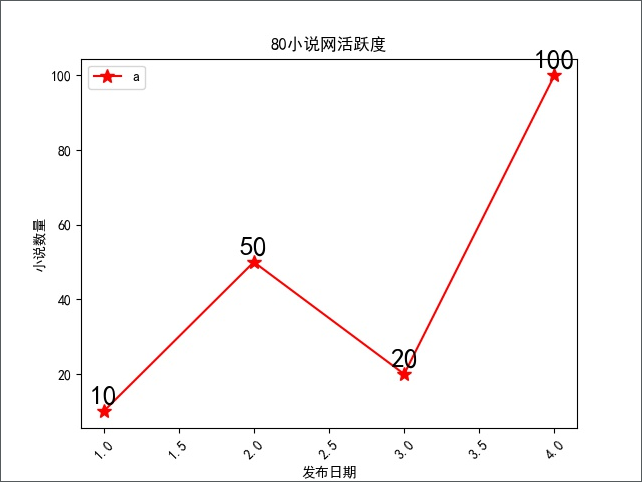注意：savefig()是图形存储成图片，show()是将图形显示出来。
绘制多条折线
# -*- coding:utf-8 -*-
import matplotlib
import matplotlib.pyplot as plt
matplotlib.rcParams['font.sans-serif'] = ['SimHei']  # 用黑体显示中文
x = [1, 2, 3, 4]
y1 = [45, 50, 20, 100]
y2 = [26, 10, 76, 25]
y3 = [11, 66, 55, 88]
y4 = [69, 50, 35, 100]
plt.plot(x, y1, marker='*', ms=10, label="a")
plt.plot(x, y2, marker='*', ms=10, label="b")
plt.plot(x, y3, marker='*', ms=10, label="c")
plt.plot(x, y4, marker='*', ms=10, label="d")
plt.xticks(rotation=45)
plt.xlabel("发布日期")
plt.ylabel("小说数量")
plt.title("80小说网活跃度")
plt.legend(loc="upper left")
# 在折线图上显示具体数值, ha参数控制水平对齐方式, va控制垂直对齐方式
for y in [y1, y2, y3, y4]:
for x1, yy in zip(x, y):
plt.text(x1, yy + 1, str(yy), ha='center', va='bottom', fontsize=20, rotation=0)
plt.savefig("a.jpg")
plt.show()

图形效果展示：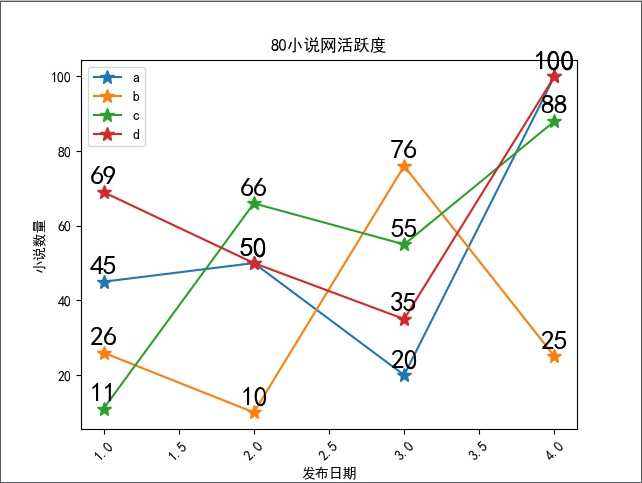matplotlib绘制柱状图
绘制普通柱状图
# -*- coding:utf-8 -*-
import matplotlib
import matplotlib.pyplot as plt
matplotlib.rcParams['font.sans-serif'] = ['SimHei']  # 用黑体显示中文
# 构建数据
x = [1, 2, 3, 4]
y = [450, 500, 200, 1000]
# 绘图
plt.bar(x=x, height=y, label='书库大全', color='steelblue', alpha=0.8)
# 在柱状图上显示具体数值, ha参数控制水平对齐方式, va控制垂直对齐方式
for x1, yy in zip(x, y):
plt.text(x1, yy + 1, str(yy), ha='center', va='bottom', fontsize=20, rotation=0)
# 设置标题
plt.title("80小说网活跃度")
# 为两条坐标轴设置名称
plt.xlabel("发布日期")
plt.ylabel("小说数量")
# 显示图例
plt.legend()
plt.savefig("a.jpg")
plt.show()

图形效果展示：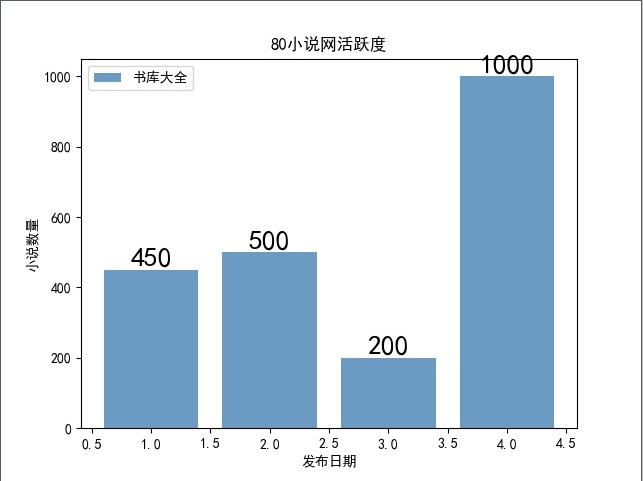绘制多组柱状图
# -*- coding:utf-8 -*-
import matplotlib
import matplotlib.pyplot as plt
matplotlib.rcParams['font.sans-serif'] = ['SimHei']  # 用黑体显示中文
# 构建数据
x = ['2015', '2016', '2017', '2018', '2019']
y1 = [4500, 5000, 2000, 7000, 10000]
y2 = [5200, 7000, 5000, 9000, 11000]
# 绘图
plt.bar(x=x, height=y1, label='python', color='steelblue', alpha=0.8)
plt.bar(x=x, height=y2, label='java', color='indianred', alpha=0.8)
# 在柱状图上显示具体数值, ha参数控制水平对齐方式, va控制垂直对齐方式
for x1, yy in zip(x, y1):
plt.text(x1, yy + 1, str(yy), ha='center', va='bottom', fontsize=20, rotation=0)
for x1, yy in zip(x, y2):
plt.text(x1, yy + 1, str(yy), ha='center', va='bottom', fontsize=20, rotation=0)
# 设置标题
plt.title("python与java图书对比")
# 为两条坐标轴设置名称
plt.xlabel("年份")
plt.ylabel("销量")
# 显示图例
plt.legend()
plt.savefig("a.jpg")
plt.show()

图形效果展示：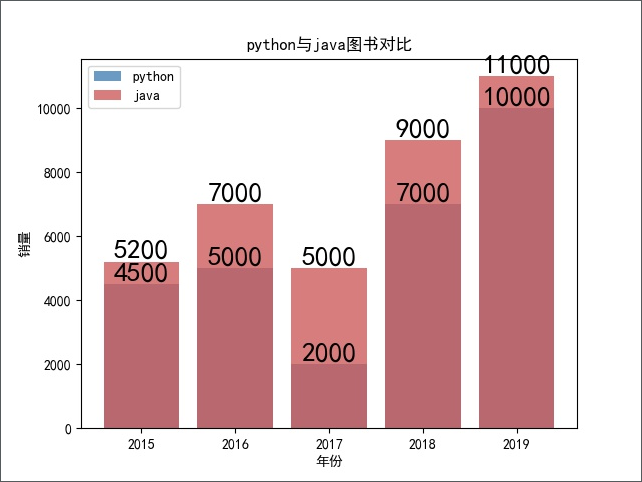绘制柱状图的条柱并列显示
# -*- coding:utf-8 -*-
import numpy as np
import matplotlib
import matplotlib.pyplot as plt

matplotlib.rcParams['font.sans-serif'] = ['SimHei']  # 用黑体显示中文
# 构建数据
x = ['2015', '2016', '2017', '2018', '2019']
y1 = [4500, 5000, 2000, 7000, 10000]
y2 = [5200, 7000, 5000, 9000, 11000]
bar_width = 0.3
# 将X轴数据改为使用range(len(x_data), 就是0、1、2...
plt.bar(x=range(len(x)), height=y1, label='python', color='steelblue', alpha=0.8, width=bar_width)
# 将X轴数据改为使用np.arange(len(x_data))+bar_width,
# 就是bar_width、1+bar_width、2+bar_width...这样就和第一个柱状图并列了
plt.bar(x=np.arange(len(x)) + bar_width, height=y2, label='java', color='indianred', alpha=0.8, width=bar_width)
# 在柱状图上显示具体数值, ha参数控制水平对齐方式, va控制垂直对齐方式
for x1, yy in enumerate(y1):
plt.text(x1, yy + 1, str(yy), ha='center', va='bottom', fontsize=20, rotation=0)
for x1, yy in enumerate(y2):
plt.text(x1 + bar_width, yy + 1, str(yy), ha='center', va='bottom', fontsize=20, rotation=0)
# 设置标题
plt.title("python与java对比")
# 为两条坐标轴设置名称
plt.xlabel("年份")
plt.ylabel("销量")
# 显示图例
plt.legend()
plt.savefig("a.jpg")
plt.show()

图形效果展示：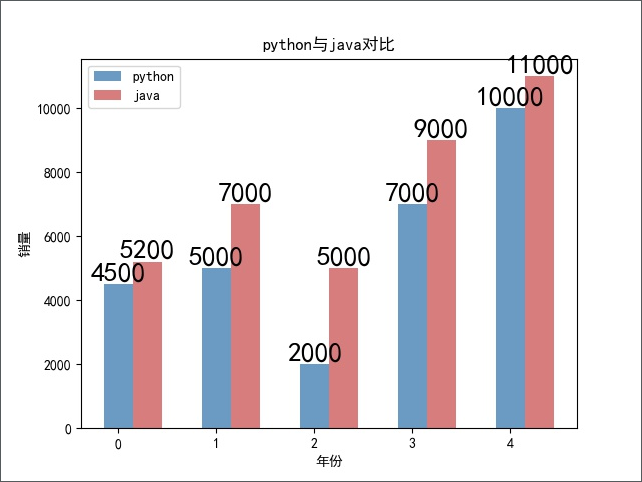matplotlib绘制柱线混合图
绘制柱线混合图
# -*- coding:utf-8 -*-
import matplotlib
import matplotlib.pyplot as plt
matplotlib.rcParams['font.sans-serif'] = ['SimHei']  # 用黑体显示中文
# 构建数据
x = [2, 4, 6, 8]
y = [450, 500, 200, 1000]
# 绘图
plt.bar(x=x, height=y, label='书库大全', color='steelblue', alpha=0.8)
# 在柱状图上显示具体数值, ha参数控制水平对齐方式, va控制垂直对齐方式
for x1, yy in zip(x, y):
plt.text(x1, yy + 1, str(yy), ha='center', va='bottom', fontsize=20, rotation=0)
# 设置标题
plt.title("80小说网活跃度")
# 为两条坐标轴设置名称
plt.xlabel("发布日期")
plt.ylabel("小说数量")
# 显示图例
plt.legend()
# 画折线图
plt.plot(x, y, "r", marker='*', ms=10, label="a")
plt.xticks(rotation=45)
plt.legend(loc="upper left")
plt.savefig("a.jpg")
plt.show()

图形效果展示：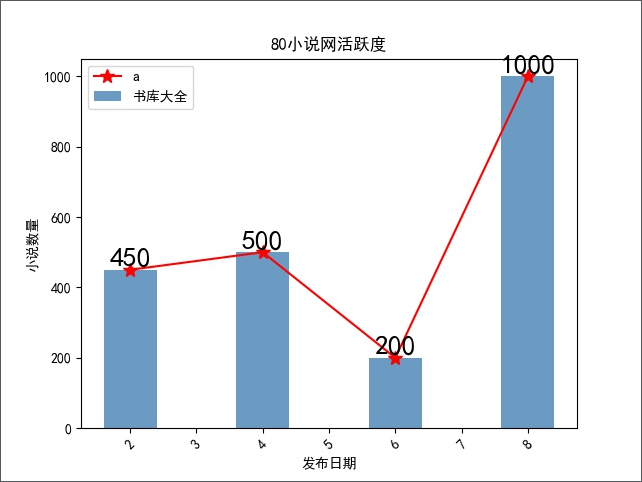展开全文matplotlib python
• 所谓对比分析就是两个相互联系的指标进行比较 下面用例子说明，首先导入库，别名 因为我用的是jupyter notebook，后面需要用matplotlib画图，所以要加上%matplotlib inline import pandas as pd import nummpy ...
所谓对比分析就是两个相互联系的指标进行比较
下面用例子说明，首先导入库，别名
因为我用的是jupyter notebook，后面需要用matplotlib画图，所以要加上%matplotlib inline
import pandas as pd
import nummpy as np
import matplotlib.pyplot as plt
from IPython.display import display
plt.style.use('fivethirtyeight') #设置图像风格样式
import seaborn as sns
sns.set_style({'font.sans-serif':['simhei','Arial']}) #设置字体样式，防止后面画图时中文字体显示出现问题
%matplotlib inline 
这里先随机生成一组30x2的数据，大概1000左右量级
data=pd.DataFrame(np.random.rand(30,2)*1000,#这里表示随机生成30行数据，有两列，1000左右的量级
columns=['A_sale','B_sale'],
index=pd.period_range('2019-8-1','2019-8-30'))
data
查看前10行，看看随机生成的数据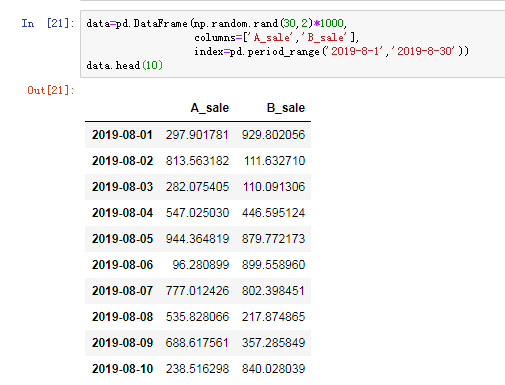现在来绘制AB产品销量的折线图
data.plot(kind='line',
style='--o',
figsize=(20,5),
title='AB产品销量对比---折线图')
画出的图形如下：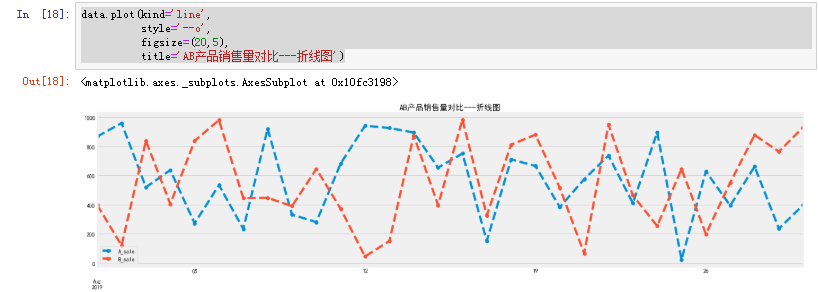试试柱状图，把代码kind='line'中的‘line’改成‘bar’,去掉线的样式就行了
data.plot(kind='bar',
figsize=(20,5),
title='AB产品销量对比---柱状图')
效果如下：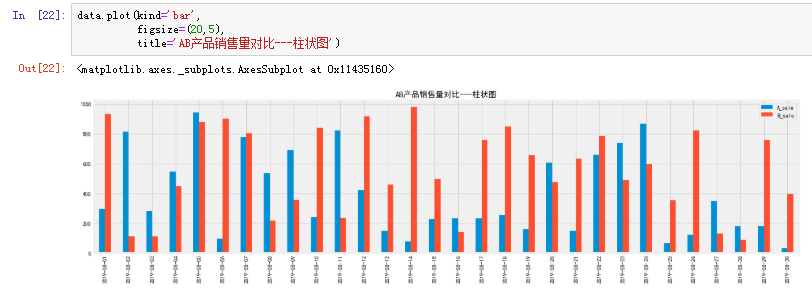如果要看AB产品销量之间的差值，那就可以先算出AB销量之间的差值，再进行可视化
下面代码利用plt.axhline()设置了参考线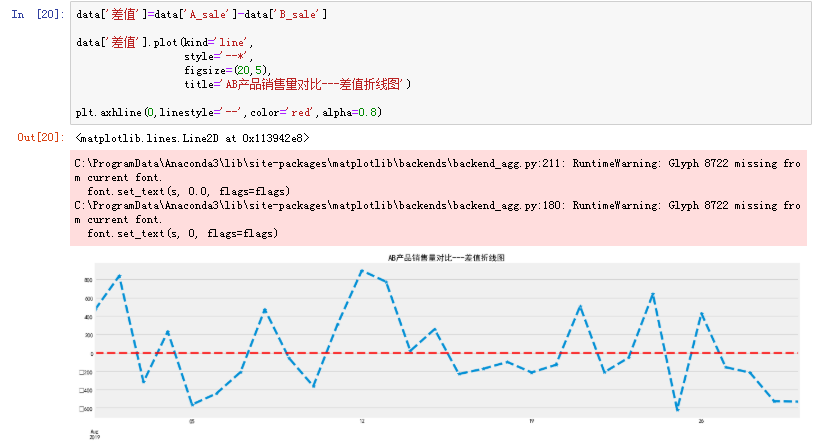展开全文• Web统计图表插件示例，包含饼状图、柱状图折线图等；多种表现形式，分类、合并、对比等图表均有
• 图表 数据分析工作，离不开图表的展示。有时候表展示的数据更加清晰，有时候...比如这次遇到这样的需求：分析一下2020年数据与2019年同期数据的对比分析工作，数据大概如下，一列日期，一列数据。（案例数据用excel
• Python在一些数据可视化的过程中需要使用 plt 函数画柱状图和折线图。 2. 导入 import matplotlib.pyplot as plt 3. 柱状图 array= np.array(array) plt.hist(array, bins=50,facecolor="red", ...
• 根据excel内容画折线图和柱状图，具体可参考：https://blog.csdn.net/u010637291/article/details/117189249（其对应的代码和数据）matlab
• Excel图表的作用非常重要，...如上图所示，我们需要通过每款产品的销量转化率做柱状图来展示数据对比。因为销量是百分数，转化率是百分比，所以数据维度上面两组数据相差很大。通过这两组差异数据如果只是做柱状图...
• 折线图一枚 \$("#z_line").highcharts({ chart: { type: 'line' }, credits: { enabled: false // 禁用版权信息 }, ti...
• 对比分析图表在工作中应用最普遍也最广泛，销售分析、费用对比、年度统计等都少不了，最常用的对比分析图表是柱形图和条形图。今天就和大家分享这两种最基础的对比分析图，看它们的千秋纷呈。柱形图柱状图最常见，它...python matplotlib pyplot
• 利用MATLAB同时绘制柱状图和折线图，其中柱状图表示温度，折线图表示颗粒物浓度，左侧y轴表示温度（单位摄氏度），右侧y轴表示颗粒物浓度（个/每立方米）。MATLABplotyy方法/步骤第一，以某地区一周的温度和空气中...
• 柱状图Bar，折线图Line是最常用的图形，本节来介绍如何创建它们。 1.柱状图的创建： （1）纵向柱状图 使用Bar可以轻松创建一个柱状图，例子代码如下： from pyecharts.charts import Bar from pyecharts import...pyecharts python Bar
• 我们首先来看看在绘制barplot（柱状图）时，可能会不知所措的一些麻烦，我们主要通过一段示例代码来实现下面四个功能（其中加标签的方法是比较tricky的），最终绘制出来的效果图如下图所示。 1）为柱状图中的每...
• 相信很多小伙伴都已经知道如何建立Excel柱形图，但对于在excel柱形图上添加折线图大家又会不会呢？在excel柱形图上添加折线图能让我们的图表更加清晰，而且这还是一个提问率非常高的Excel图表问题，今天小编就以实例...
• 问题1：横向的柱状图 xAxis : [ { type : 'value' } ], yAxis : [ { type : 'category', name: '幢', ...
• 柱状图表示各区的在线率，同时显示一个折线，表示全市的平均值， 用于各区与全市平均的对比，是高于平均还是低于平均。 去官网搜了下，混合图表，也能满足我的需求，但有些差别， 混合图双坐标代表的是两种不同的....net c#
• 本代码是一年前公司项目需要自己所写,当时做android项目需要统计的功能，统计的图表包括饼图、柱状图、...此含有柱状图和折线图的组合，有兴趣的可以拆分成单个的折线图和柱状图。 LineHistogram.java package comandroid
• 关于matplotlib的学习 plt.xticks() 矢量图设置 柱状图-适合多种算法对比
• itextpdf 添加折线图、饼图、柱状图 时隔三年，又要对PDF一顿改版了，此次改版加入了饼图、折线图柱状图等图形可视化。 文章目录itextpdf 添加折线图、饼图、柱状图依赖包效果图步骤生成折线图生成柱状图生成...数据可视化 itext jfreechart
• Pygal 同样支持各种不同的数据图，比如饼图、折线图等。...折线图柱状图很像，它们只是表现数据的方式不同，柱状图使用条柱代表数据，而折线图则使用折线点来代表数据。因此，生成折线图的方式与生成柱...python
• 本文以完成课程作业为基础，利用matplotlib生成扇形图、折线图柱状图、等值线图和3D绘图python 可视化
•  /// 目前能够画2维图3维图 柱形图 折线图 饼形图。韩永矗5Apr2005制作。  /// 柱形图请将xml文件中的“add key="DiagramType"”修改为histogram  /// 折线图请将xml文件中的“add key="DiagramType"”修改...string components xml null
• 首先在build.gradle 里面添加依赖 compile ‘com.github.PhilJay:MPAndroidChart:v3.0.1’ 然后在布局里引用想展示的图表类型柱状图 android:id="@+id/barChart1" android:layout_w布局 class...